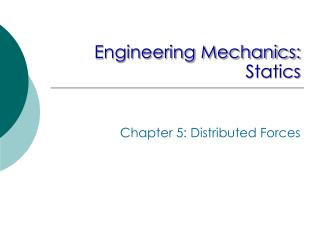DownloadDownload PresentationEngineering Mechanics: Statics

# Engineering Mechanics: Statics

Télécharger la présentation## Engineering Mechanics: Statics

- - - - - - - - - - - - - - - - - - - - - - - - - - - E N D - - - - - - - - - - - - - - - - - - - - - - - - - - -
##### Presentation Transcript

1. Engineering Mechanics: Statics Chapter 5: Distributed Forces

2. Introduction ‘Concentrated’ force does not exist, since every force applied mechanically is distributed over a finite contact area. When the applied region is not negligible, we must account for the actual manner the force is distributed Three categories: Line distribution (N/m) Area distribution (N/m2, Pa) Volume distribution or body force (N/m3)

3. Centers of Mass Body force due to gravitational attraction of the earth (weight) is the most commonly encountered distributed force This section treats the determination of the point in a body through which the resultant gravitational force acts. Center of gravity Apply the principle of moments (The moment of the resultant force W equal the sum of the moments about the same axis of the force dW acting on all particles) Center of mass

4. Centers of Massand Centroids In vector form where and The density r of a body is its mass per unit volume, thus , etc. • When the density r of a body is uniform throughout, the expressions define purely geometrical property of the body and the term centroid is used.

5. Centroids • Centroid of lines • Centroid of areas • Centroid of volumes • In a homogeneous body, center of mass always lies on a plane of symmetry! • Since the moments due to symmetrically located elements will always cancel.

6. Choice of Element for Integration • Order of element • Continuity • Discard higher-order term (integration of ydx instead of ydx+1/2dydx) • Choice of coordinate system • Centroidal coordinate of element

7. Sample Problem 5/2 Determine the distance from the base of a triangle of altitude h to the centroid of its area

8. Sample Problem 5/5 Locate the centroid of the volume of a hemisphere of radius r with respect to its base.

9. Composite Bodies • When a body can be divided into several parts whose mass centers are easily determined, we use the principle of moments and treat each part as a finite element of the whole

10. Sample Problem 5/6 Locate the centroid of the shaded area. 120 40 40 30 20 50 Dimensions in mm 20 20 30

11. Problem 5/57 The rigidly connected unit consists of a 2-kg circular disk, a 1.5-kg round shaft and a 1-kg square plate. Determine the z-coordinate of the mass center of the unit.